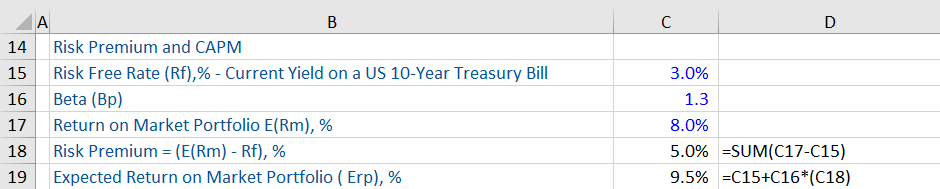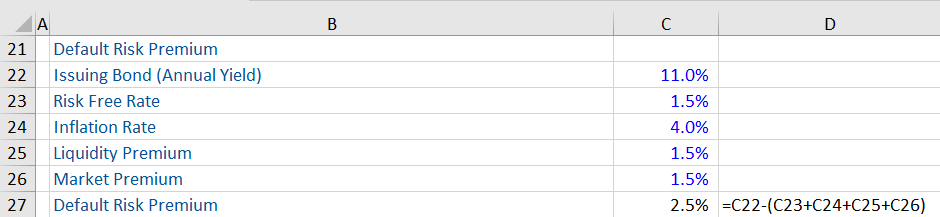## What are “Investment Risks?”

Investments of any type are exposed to some degree of investment risk. When investors invest in stocks, corporate bonds, real estate or other types of investments, they are exposed to various risks that can adversely impact their returns on investment. These risks, termed ‘investment risks’, are defined as the probability of decline in value (rather than profit) of an investment or investments due to fall in the fair price of the same. This may be as a result of economic developments or other events that adversely impact the entire market.

Various types of risks can affect investments including: market risk (includes equity, interest rate and currency risks), liquidity risk, credit risk, inflation risk, reinvestment risk, foreign investment risk, horizon risk, sovereign risk, real interest rate risk, longevity risk and concentration risk. Investment risks can be managed through the diversification of investments and investing for the long-term.

## Key Learning Points

• Investments of any type are exposed to some degree of risk
• The relationship between risk and returns infers that for exposure to higher potential returns, and investor must be willing to accept increased investment risks
• There are various types of investment risk which can impact investment strategies
• With reference to stocks, market risk refers to systematic risk and there are two related concepts – market risk premium and CAPM
• Default risk is a subcategory of credit risk, which is applicable to all bonds
• Default risk premium is the extra interest that is paid by a borrower (as compensation to the lender) for assuming risk of default on bond obligations.

## Investment Risks – Risk Premium, CAPM and Default Risk

While there are many investment avenues, the focus here is on risk associated with equities and bonds. With stocks, there is a type of investment risk known as market risk which is the systematic risk or the risk that is inherent in the entire market. There are two related concepts – Risk Premium or Market Risk Premium and Capital Asset Pricing Model (CAPM) – that should be known before undertaking investment strategies.

“Risk Premium” or “Market risk Premium” is defined as the rate of return on an investment which is in excess or over and above the risk-free rate (e.g. a US Treasury bill). This is what an investor seeks to be compensated for from investing in high-risk investments. The higher the investment risk, the higher the risk premium is likely to be. This can be stated as:

• Risk Premium = Return on Investment on a Stock – Risk Free Return on US Treasury Bills
• Market Risk Premium = Average Market Return (Rm) i.e. return on a whole stock index such as S&P 500) – Risk Free Return (Rf)

Next, the risk premium on a stock or equity using the CAPM is beta multiplied by the difference between the market rate of return and the risk-free rate of return.

• E(Rp) = Rf + Bp * (E(Rm) – Rf)
• E(Rp) = expected return on equity
• Rf = risk free rate
• Bp = Beta of the investment (measures the systematic risk)
• (E(Rm) – Rf) = “Risk Premium.”

Next, credit risk is inherent in all bonds and one of the categories of such risk is the default risk. Related to this is the concept of default risk premium. This is the additional amount of interest paid by a borrower as compensation for assuming default risk.

Default Risk Premium = Total Interest Charged on a Bond – Other Components of Interest (Risk Free Rate + Inflation Rate + Liquidity Premium + Maturity Premium)

## Investment Risks – Examples

Given below are examples (2) of risk premium and default risk premium.

In the first example, assume that an investor is planning to invest \$60,000, in order to get a high return on investment. This investor is well aware of the potential risks of investments and wants to balance the risk-return trade-off vis-à-vis the same. The investor has the option of investing in a risk free asset such as the US treasury bond that returns 3% a year. The other option is to invest in a metal stock, which has the potential of giving 22% returns.

In order to obtain this investor’s risk premium (\$11,400), we first calculate the risk-free return (\$1,800) and then the return on investment (\$13,200) on this stock. By taking the difference between the two we get the risk premium. Further, note that this investor’s risk premium is an approximate estimate of the expected returns on the perceived risky asset i.e. on the metal stock so returns are not guaranteed.Given below is an example of the calculation of  Risk Premium i.e. Market Risk Premium (E(Rm – Rf) and Expected Return on Equity (Erp) – using CAPM – for the US market. The risk premium is 5%.Next, assume that Company A is issuing bonds with an annual percentage yield of 11% (i.e. total interest charged is 11%). The default risk premium is calculated below (using the aforesaid formula).## Conclusion

It is important to identify all possible investment risks before investing, bearing in mind that the higher the potential returns, the riskier the investment is perceived to be. The lowest risk investments available tend to be government issued bonds (from stable countries).

There is a breadth of investment risks which can all be quantified with the appropriate pre-investment analysis including: Market risk, liquidity, concentration, credit, inflation, horizon, longevity, FX and foreign investment risk. These are largely macro and economic factors which are relevant to the markets where businesses operate, the markets where the assets trade, and the more specific risks undertaken by the individual investment as part of their overall investment strategy. Investment risks can be managed through the diversification of investments and investing for the long-term.